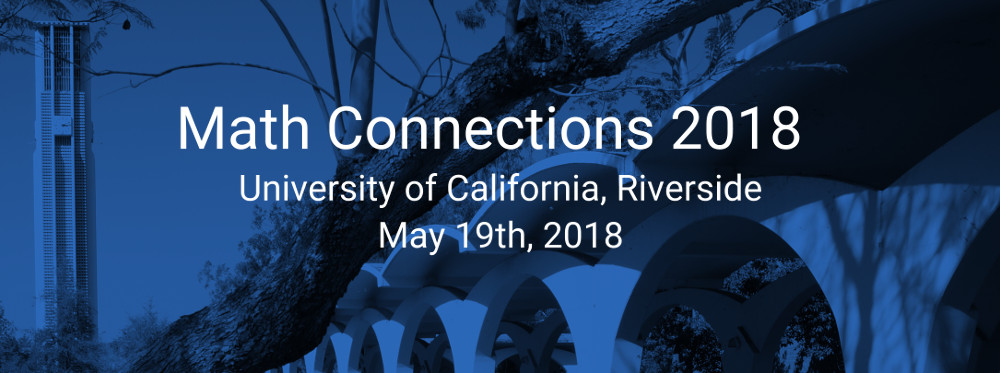#### Program

The conference will have several sessions of 25 minute talks given by graduate students; coffee, snacks, and lunch will be provided. The conference will finish with a plenary talk given by professor Michel L. Lapidus (UCR). The schedule can be found below, and you can download a pdf version here:

 Time/Rooms Surge 284 Surge 268 9:30-10:30 am Reception Reception 10:30 - 11:00 am Andrea Arauza Can Ozan Oguz 11:15 - 11:45 am Kyle Castro Alexander M. Henderson 11:45 - 1:00 pm Lunch Lunch 1:00 - 1:30 pm Tim Cobler Matt O'Dell 1:45 - 2:15 pm Khoi Vo Gene B. Kim 2:30 - 4:00 pm Plenary Talk -----

### Can One Hear the Shape of a Fractal Drum?

#### The Sound of Fractal Strings and the Riemann Hypothesis

##### by Dr. Michel L. Lapidus, Distinguished Professor of Mathematics at UCR

A well-known problem in mathematics and physics consists in understanding how the geometry (or shape) of a musical instrument affects it sound. This gives rise to two related types of mathematical problems: direct spectral problems (how the shape of a drum affects its sound) and inverse spectral problems (how one can recover the shape of a drum from its sound).

Here, we will consider both types of problems in the context of drums with fractal (that is, very rough) boundary. We will show, in particular, that one can hear the fractal dimension of the boundary (a certain measure of its roughness) and in certain cases, a fractal analog of its length. In the special case of vibrating fractal strings (the one-dimensional situation), we will show that the corresponding inverse spectral problem is intimately connected with the Riemann Hypothesis, which is arguably the most famous open problem in mathematics and whose solution will likely unlock deep secrets about the prime numbers.

In conclusion, we will briefly explain how this work eventually gave rise to a mathematical theory of complex fractal dimensions, which captures the vibrations that are intrinsic to both fractal geometries and the prime numbers.

### Parallel Session Speakers

• #### Andrea Arauza (UCR)

##### Abstract:
Noncommutative fractal geometry is the study of fractal geometry using operator algebraic tools. Our main toolkit is called a spectral triple and consists of a $C^\ast$ algebra, a Hilbert space, and an unbounded operator. Together these tools can help formulate algebraic versions of geometric concepts like dimension, geodesic distance, and measure. We will use the Sierpinski gasket and the Stretched Sierpinski gasket as our main examples.

• #### Kyle Castro (UCR)

##### Abstract:

In 1831, Peter Gustav Lejeune Dirichlet introduced the notion of a Dirichlet Character as a tool for proving the existence of quadratic nonresidues in an interval of a fixed length.  In this talk I will introduce multiplicative character sums and discuss their existing bounds.  If time permits, I will also mention the most common techniques for obtaining upper bounds on these multiplicative character sums. The non-trivial bounds being discussed continue to have applications to various problems in Analytic Number Theory and Combinatorics today.

• #### Tim Cobler (Fullerton College)

##### Abstract:
This talk will give a brief overview of what an elliptic curve is as well as some of the important related definitions and properties. It will also include a discussion of how the Birch and Swinnerton-Dyer conjecture connects the fields of algebra and analysis in studying these objects.

• #### Alexander M. Henderson (UCR)

##### Abstract:
The higher dimensional theory of complex dimensions developed by Lapidus, Radunović, and ubrinić provides a language for quantifying the oscillatory behaviour of the geometry of subsets of $\mathbb{R}^n$.  In this talk, we will describe how the theory can be extended to metric measure spaces that meet certain homogeneity conditions.  We will provide examples from $p$-adic spaces and discuss the geometric information that can be recovered from the complex dimensions in these cases.
• #### Gene B. Kim (USC)

##### Abstract:
Recall that a permutation $\pi \in S_n$ has a descent at position $i$ if $\pi(i+1) > \pi(i)$, and the descent number of $\pi$, $d(\pi)$, is the number of positions $i$ for which $\pi$ has a descent at position $i$. It is well known that the distribution of $d(\pi)$ in $S_n$ is asymptotically normal. In this talk, we discuss the distribution of $d(\pi)$ of a specific conjugacy class of $S_n$: the fixed point free involutions (otherwise known as matchings). We also explore an interesting bijection that we discovered.
• #### Matt O'Dell (UCR)

##### Abstract:
Stanley-Reisner theory provides a link between combinatorics and commutative algebra.  More specifically, this theory gives a correspondence between abstract simplicial complexes and a family of ideals in a polynomial ring.  On the other hand, Borel-de Siebenthal theory classifies the maximal semisimple subalgebras of a simple Lie algebra.  This result can be rephrased in terms of automorphisms of the simple Lie algebra and the subalgebra of fixed points.

In this talk, we give a connection between Stanley-Reisner theory and Borel-de Siebenthal theory by studying a family of bimodules for the Lie algebra of fixed points and the Stanley-Reisner ring.

• #### Can Ozan Oguz (USC)

##### Abstract:
Heisenberg algebra $\mathfrak{h}$ is one of the simplest non-commutative algebras, important in quantum mechanical systems. W-algebra $W_{1+\infty}$ is a vast extension of $\mathfrak{h}$, containing $\mathfrak{h}$ in its filtration degree zero.

We describe a diagrammatic construction of W-algebra starting from $\mathfrak{h}$, using the representation theory of the symmetric group and its categorification. This is joint work with Mike Reeks.
• #### Khoi Vo (CSU Long Beach)

##### Abstract:
The question of the density of the set of solitary numbers has been left open for a long time. It has been conjectured that its value is zero. Recently, in his paper published in 2015,  Dr. Loomis has brought up new sub-classes of solitary numbers.  In this presentation, we will proceed in proving the density of these sub-classes of solitary numbers are indeed all zero. This result helped us one step further on the way of proving the conjecture.